# Tour:Understanding the definition of a homomorphism

PREVIOUS: Equivalence of definitions of group homomorphism| UP: Introduction five (beginners)| NEXT: Equivalence of definitions of group action
General instructions for the tour | Pedagogical notes for the tour | Pedagogical notes for this part
WHAT YOU NEED TO DO: Read and enjoy the survey article about homomorphisms. Try to understand the different senses in which this notion is introduced.

The definition of a homomorphism of groups, while simple and straightforward, can seem confusing and unmotivated to people encountering it for the first time. An isomorphism of groups is a more easily understood concept: a bijection between groups that transforms the multiplication table of one to the multiplication table of the other. A homomorphism, on the other hand, far from identifies one group with the other, and the two groups could have remarkably different structure. So why study homomorphisms?

## Homomorphisms: injective, surjective and bijective

### Injective homomorphisms are subgroups

Given an injective homomorphism$\varphi:G \to H$, we can identify$G$ with its image$\varphi(G)$, which is a subgroup of$H$. Specifically, if we think of$\varphi: G \to \varphi(G)$, then it is an isomorphism, so$G$ can be identified with the subgroup$\varphi(G)$ of$H$.

Conversely, any subgroup can be viewed as an injective homomorphism: take the inclusion map.

While it is usually more useful to think of subgroups than of injective homomorphisms, sometimes it helps to view subgroups as injective homomorphisms. One advantage of this is that it intuitively makes it easier to compose with other homomorphisms, as well as helps us generalize to situations where the homomorphism is not necessarily injective. It also helps overcome the trap of thinking of a subgroup merely as a subset.

### Every homomorphism breaks up naturally as a surjective homomorphism followed by an injective homomorphism

Given a homomorphism$\varphi:G \to H$, we can break up$\varphi$ as a composite of two maps: the map$\varphi_0:G \to \varphi(G)$, which is just$\varphi$ viewed as a map only to its image, and the map$i: \varphi(G) \to H$, which is an inclusion of the subgroup in the group. Thus$\varphi = i \circ \varphi_0$, where$\varphi_0$ is surjective and$i$ is injective. Moreover, any breakup as a composite is essentially equivalent to this breakup.

Thus, studying arbitrary homomorphisms between abstract groups reduces largely to the study of injective homomorphisms and surjective homomorphisms. Both kinds of homomorphism have special behaviour. A general pointer is as follows:

1. For an injective homomorphism (or a subgroup): The group on the right (i.e., the big group) controls, to some extent, the behaviour of the group on the left. There is very little reverse control.
2. For a surjective homomorphism (or a quotient map): The group on the left controls, to some extent, the behaviour of the group on the right. There is very little reverse control.

(It's also true that every homomorphism breaks up as an injective homomorphism followed by a surjective homomorphism, but this breakup is not natural, and is not important for understanding structure).

## Homomorphisms from a universal algebraic perspective

Most of the facts stated here are of a universal algebra-flavour: they work in the generality of any variety of algebras.

### Preserves words

A map$\varphi:G \to H$ is termed a homomorphism of groups if$\varphi(e) = e$,$\varphi(g^{-1}) = \varphi(g)^{-1}$ for all$g \in G$, and$\varphi(g_1g_2) = \varphi(g_1)\varphi(g_2)$ for all$g_1, g_2 \in G$.

From this, we see that if$w$ is a word, then: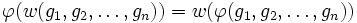$\varphi(w(g_1,g_2,\dots,g_n)) = w(\varphi(g_1,g_2,\dots,g_n))$

For instance, if we have:$w = (x_1,x_2,x_3) \mapsto x_3x_2^{-1}x_1^2x_3^2x_2$

Then:$\varphi(w(g_1,g_2,g_3)) = \varphi(g_3g_2^{-1}g_1^2g_3^2g_2) = \varphi(g_3)\varphi(g_2)^{-1}\varphi(g_1)^2\varphi(g_3)^2\varphi(g_2) = w(\varphi(g_1),\varphi(g_2),\varphi(g_3))$

### Preserves equations

Since homomorphisms preserve words, they also preserve equations. So, if we have, for some particular word$w$ and some specific choice of$g_1,g_2,\dots,g_n \in G$:$w(g_1,g_2,\dots,g_n) = w'(g_1,g_2,\dots,g_n)$

Then, under the homomorphism$\varphi: G \to H$:$w(\varphi(g_1),\varphi(g_2),\dots,\varphi(g_n)) = w'(\varphi(g_1),\varphi(g_2),\dots,\varphi(g_n))$

In particular, this means that solutions to equations in$G$ give solutions to equations in$H$.

### Don't preserve inequations, unless injective

Intuitively, we can think of a homomorphism as a collapse. Whatever equations hold in$G$ for elements of$G$, hold for their images in$H$. However, the situation becomes markedly different when we're looking at inequations. In other words:$g_1 \ne g_2 \not \implies \varphi(g_1) \ne \varphi(g_2)$

Thus, for instance, if we can solve a system of equations for variables$g_1,g_2$ in$G$ under the constraint that$g_1 \ne g_2$, then we can tkae the images of these solutions to solve the equations in$H$. But it could well happen that the images of the solutions are equal.

As an extreme example, consider the trivial group$\{ e \}$ and the homomorphism sending$G$ to$\{ e \}$. Then, under this homomorphism, all elements of$G$ become equal in the image.

There is a special case where the homomorphism preserves both equations and inequations: namely, when it is an injective homomorphism. An injective homomorphism$\varphi:G \to H$ can be viewed as embedding$G$ as a subgroup of$H$ (the subgroup being its image$\varphi(G)$).

### Don't preserve universal identities, unless surjective

Intuitively, given a homomorphism$\varphi:G \to H$, the only control we have on$H$ using$G$, is on the subgroup$\varphi(G)$ of$H$. Elements outside the image of$\varphi$ could behave in ways completely uncontrolled by$G$.

When the map$\varphi:G \to H$ is a surjective homomorphism (and hence, is equivalent to a quotient map), then if we have a universal identity on$G$, i.e. a word$w$ such that:$w(g_1,g_2,\dots,g_n) = e \ \forall g_1,g_2,\dots,g_n \in G$

Then,$w$ also gives a universal identity on$H$. That's because all elements of$H$ can be written as$\varphi(g_i)$ for some$g_i \in G$, and we get:$w(\varphi(g_1),\varphi(g_2),\dots,\varphi(g_n)) = \varphi(w(g_1,g_2,\dots,g_n)) = \varphi(e) = e$

### Equations cannot be pulled back

Suppose$w$ is a word,$\varphi:G \to H$ is a homomorphism of groups, and we have a tuple$h_1,h_2,\dots,h_n$ such that, in$H$: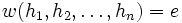$w(h_1,h_2,\dots,h_n) = e$

It may not in general be possible to find$g_i$ such that$\varphi(g_i) = h_i$ and$w(g_1,g_2,\dots,g_n) = e$. There are two obstacles:

• Since$\varphi$ is not necessarily surjective, we may not be able to find inverse images of each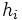$h_i$
• Even if we succeed, all that yields to us is that$\varphi(w(g_1,g_2,\dots,g_n)) = e$. In order to use this to conclude that$w(g_1,g_2,\dots,g_n) = e$, we need the map$\varphi$ to be injective.

Thus, we need the map$\varphi$ to be an isomorphism of groups to guarantee being able to pull back equations. Actually, conditions weaker than being an isomorphism suffice. For instance, if$\varphi$ is a retraction, i.e., if it is a surjective map with a one-sided inverse injective map, then solutions can always be pulled back, using the injective map. Further information: Retract, Equation-closed subgroup

WHAT'S MORE: Some more things about homomorphisms, based on ideas that you'll see later in the tour.

### Surjective homomorphisms are quotient maps

Given a surjective homomorphism$\varphi:G \to H$ with kernel$N$, we can, by the first isomorphism theorem, find a unique map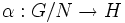$\alpha:G/N \to H$ such that if$q: G \to G/N$ is the quotient map, then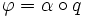$\varphi = \alpha \circ q$. In other words, any surjective homomorphism can be identified with a quotient map, and hence, with a normal subgroup (which determines the quotient map with that as kernel).

It is usually more helpful to think of quotient maps than surjective homomorphisms, but surjective homomorphisms offer some advantages: being able to compose, and escaping the trap of thinking of quotients in terms of normal subgroups and cosets.